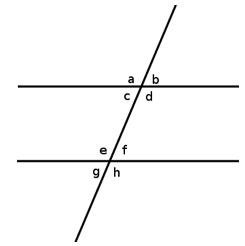# GMAT Geometry Basics: Parallel Lines

We can say that two lines are “parallel” if they never intersect and get neither closer nor farther away from one another. || is the symbol for parallel lines. In coordinate geometry, parallel lines have the same slope, but different x-intercepts.

Try this GMAT geometry practice question and test your skills today!

Parallel lines can be tested in either Problem Solving or Data Sufficiency and though the concept itself is relatively simple, the test questions presented can appear somewhat complex. Don’t make assumptions about lines that look parallel but may in fact not be. Let’s look at an example of how the “eyes can deceive” from GMATPrep:This question tells us that x < 90 and y < 90 and that PS and QR are parallel. We need to be able to answer whether or not PQ < SR. We cannot assume that PQ and SR are parallel simply because they look parallel, or that x = y. Statement (1) tell us that x > y. Since x is greater than y, then PQ will be slightly shorter (more vertical) than SR. Thus, (1) is sufficient. Statement (2) tells us that the sum of the angles is greater than 90, but does not tell us the value of each angle. It is insufficient.  Let’s try a question from Grockit:

If the two horizontal lines in the figure provided are parallel, what is (a + f + d) – (e + h)?1)  a + f = 180

2)  af = 60

This question solely relies on our knowledge of angle measures and parallel lines. Angles a and b are complementary (add up to 180, a straight line), and angles b and f are congruent, so we already know that a + f = 180 without Statement (1) telling us. (a + f + d) – (e + h) = a + f + d – e – h = 180 – h. Since we do not know the exact value of h (the difference between the obtuse and acute angles), Statement (1) is not sufficient. Statement (2) gives us the difference between the obtuse and acute angle, and so it is sufficient.

Follow me on Beat the GMAT to read more of my posts, or message me via Grockit for more help with Parallel Lines questions!

0 replies